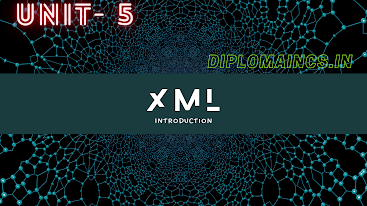## 12/21/22

### Computer Organization and Architecture 2022 previous year

#### Computer Organization and Architecture 2022 previous year

Sub Code: - 2018303

2022(Odd)

Time : 3Hrs

Semester  III/CSE

COA

Full Marks : 70

Pass marks : 28

Group A

Choose the most suitable answer from the following options: -   (1*20=20)

(i) A byte is group of _____________ bits.

(a) 16

(b) 8

(c) 4

(d) 28

(ii) The program counter is also called as :

(a) Instruction Pointer

(b) Data counter

(c) Memory pointer

(d) None of the above

(iii) Which of the following computer register collects the results of computation?

(a) Accumulator

(b) Instruction pointer

(c) Storage register

(d) None of the above

(iv) Which of the following is the operation executed on data stored in registers?

(a) Byte operation

(b)Bit operation

(c) Macro operation

(d) Micro operation

(v) Which of the following register can interact with the secondary storage?

(a) PC

(b) MAR

(c) MDR

(d) IR

(vi) (2FAOC)16 is equivalent to:

(a) (195084)10

(b) (00101111101000001100)2

(c) Both (a) and (b)

(d) None of these

(vii) Floating point representation is used to store:

(a) A Boolean value

(b) Whole number

(c) Real number

(d) Integers

(viii) The performance of cache memory is frequency measured in terms of quality called:

(a) Miss ratio

(b) Hit ratio

(c) Latency ratio

(ix) BIOS programs are embedded on chip called___________

(a) Firmware

(b) IC

(c) Hardware

(d) Application programs

(x) Which of the following is not hardware component of computer?

(a) Memory

(b) Scanner

(c) Software

(d) CPU

(xi) To use peripheral device following modules are required:

(a) I/O controller

(b) I/O deriver

(c) I/O Memory

(d) Only (a) and (b)

(xii) Depending on mechanism of timing data, data transfer can be:

(a) Synchronous

(b) Asynchronous

(c) Both (a) and (b)

(d) None of these

(xiii) Which of the following is not the correct technique to reduce cache miss rate?

(a) Compiler optimization

(b) Use of victim cache

(c) Lower associativity

(d) Use of large cache

(xiv) Where is decoded instruction stored?

(a) Register

(b) MDR

(c) PC

(d) IR

(xv) The status bit is called as:

(a)  Unsigned bit

(b) Signed bit

(c) Flag bit

(d) None of the above

(xvi) Virtual memory is consisting of:

(a) Static RAM

(b) Dynamic RAM

(c)  Magnetic memory

(d) None of these

(xvii) A stack-organized computer uses instruction of:

(xviii)  Which of the following circuit is used to store 1 bit of data?

(a)  Flip Flop

(b) Decoder

(c) Encoder

(d) Register

(xix) Which of the following operation is/are performed by the ALU?

(a)  Data manipulation

(b) Exponential

(c) Square root

(d) All of the above

(xx) Which of the following allow simultaneous write and read operations?

(a) ROM

(b) EROM

(c)  RAM

(d) None of there

Group:-"B"

Answer all Five Questions: -                         (5*4=20)

2.  Define pipelines processing with suitable examples.

OR

Explain various types of interrupts.

3.  Draw and explain the organization of micro program control unit.

OR

State and explain the rule in arithmetic operation on floating point numbers.

4. Why NAND and NOR gate is known as universal gate?

OR

Compare RISC and CISC systems?

5.  What is ROM? How does PROM differ from EEPROM?

OR

What are the advantages and disadvantages of hardwired and micro programmed control.

6. Write short note on subroutine call and return.

OR

Explain a synchronous data transfer using timing diagrams.

Group:- "C"

Answer all Five Questions: -                         (5*6=30)

7.  Write detailed note on Direct Memory Access (DMA).

OR

Explain BCD adder with its block diagram.

8. Explain booth multiplication algorithm for multiplying binary integers in signed 2’s complement representation.

OR

What is register stack? Explain push and pop micro-operations.

9. Discuss associative mapping and direct mapping in organization of cache memory.

OR

What do you mean by fetch cycle, instruction cycle, machine cycle, interrupt acknowledgement cycle?

10. What do we require memory hierarchy? Show the memory hierarchy diagram.

OR

What is virtual memory? Why is called virtual? Write the advantages of virtual memory?

11.  Explain decoder with logic circuit and truth table.

OR

What is an addressing mode? Explain any four types of addressing modes with examples?

### To access previous year question of the year 2017 to 2021